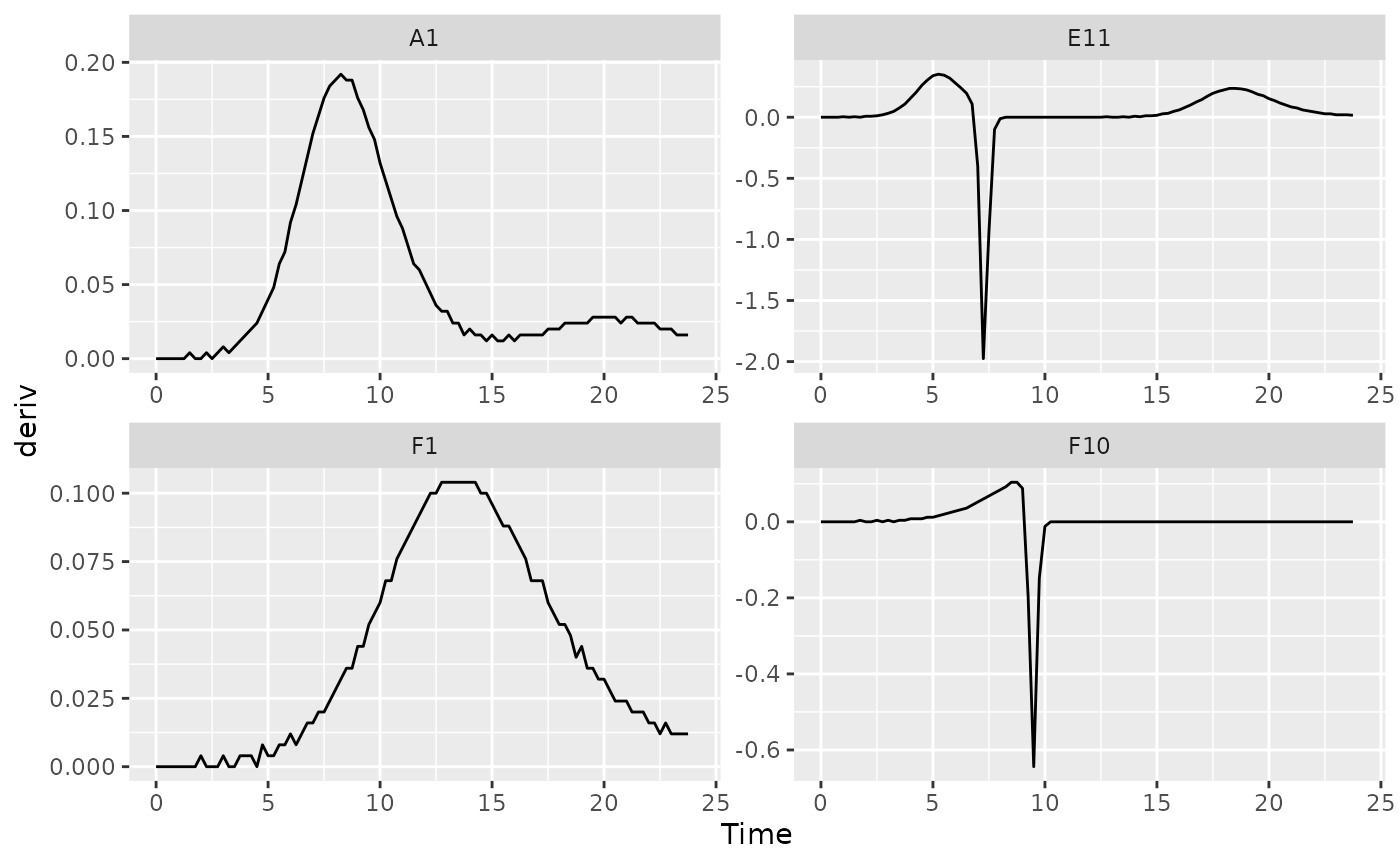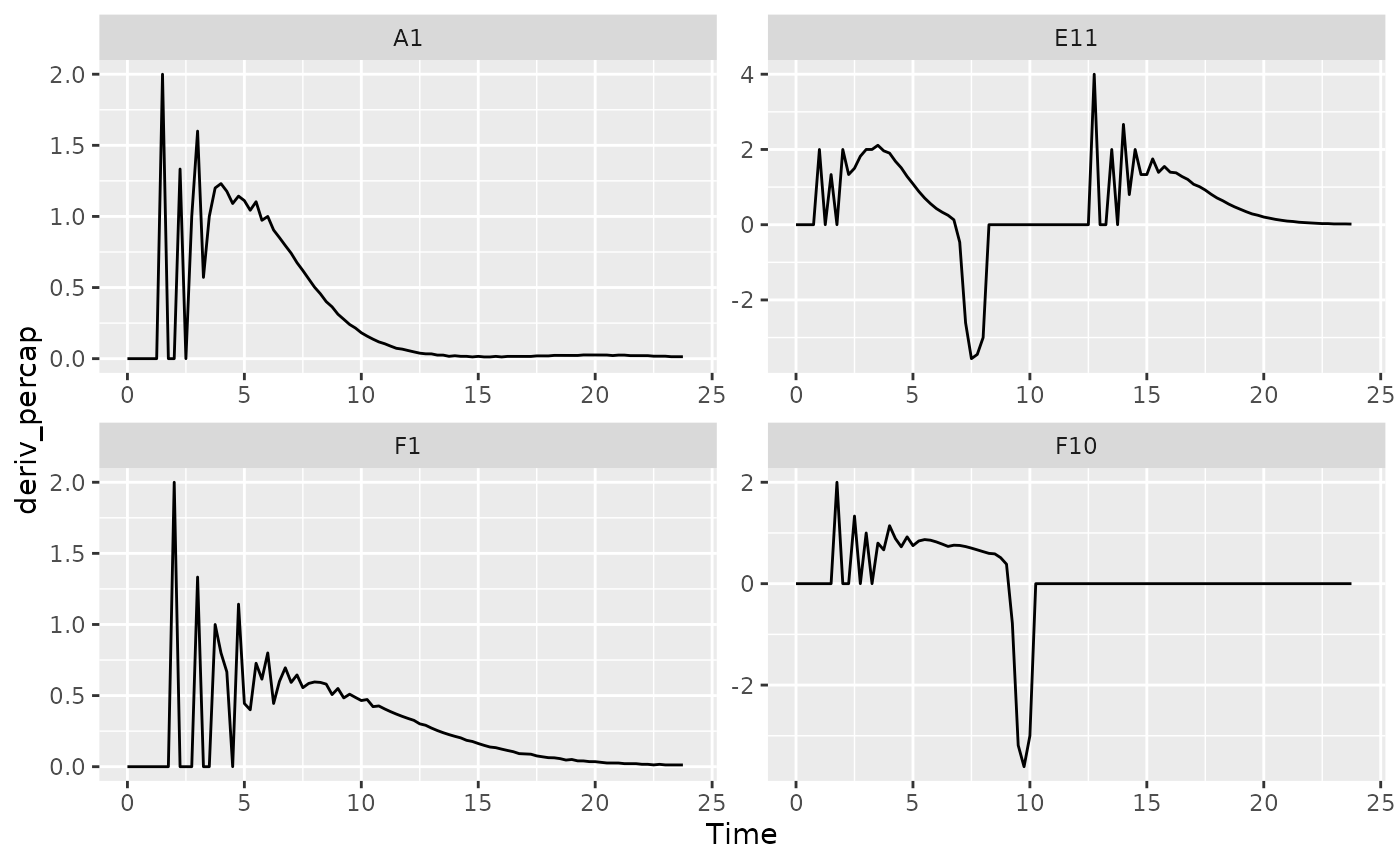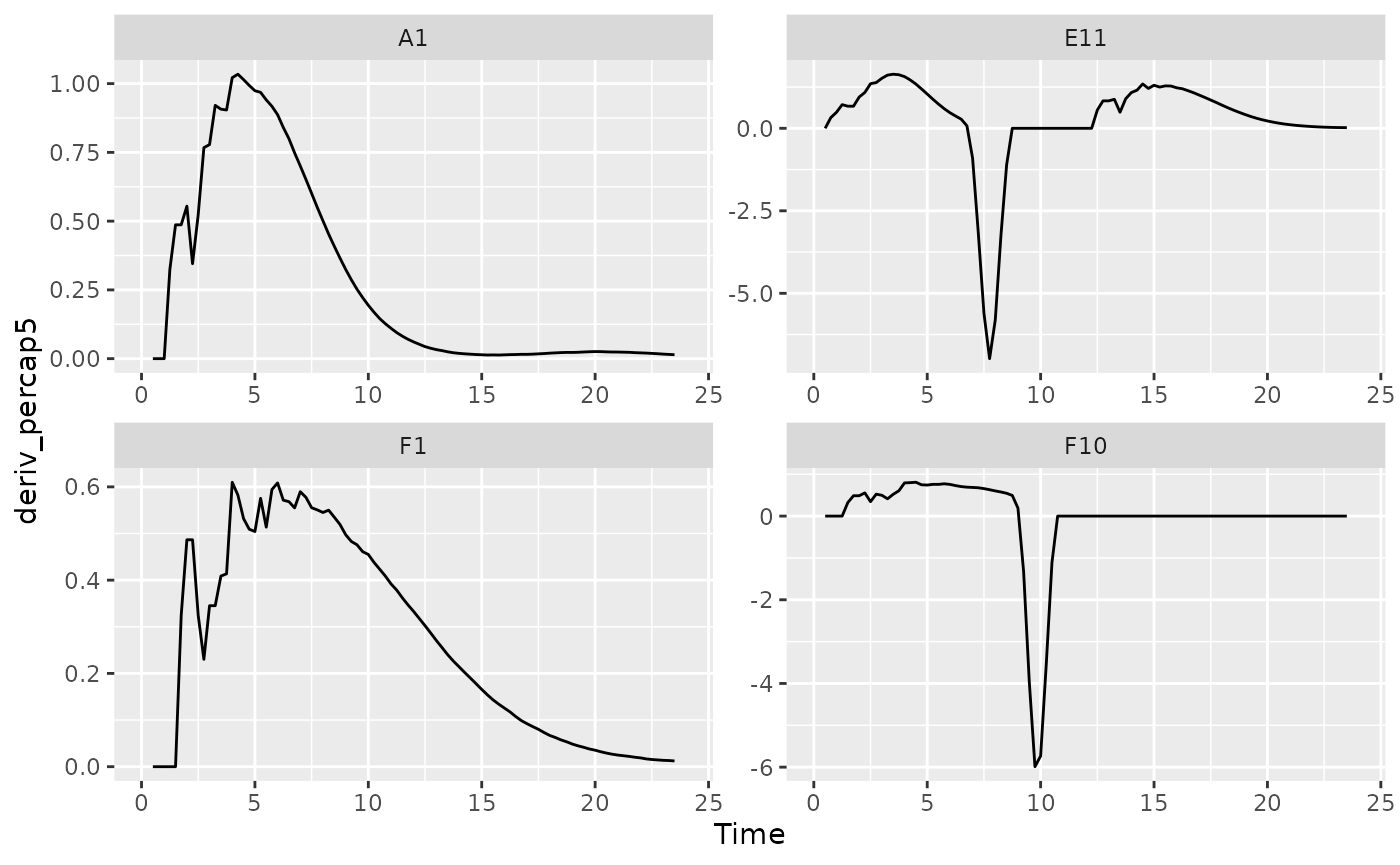## Where are we so far?

1. Introduction: vignette("gcplyr")
2. Importing and transforming data: vignette("import_transform")
3. Incorporating design information: vignette("incorporate_designs")
4. Pre-processing and plotting your data: vignette("preprocess_plot")
5. Processing your data: vignette("process")
6. Analyzing your data: vignette("analyze")
7. Dealing with noise: vignette("noise")
8. Statistics, merging other data, and other resources: vignette("conclusion")

So far, we’ve imported and transformed our measures, combined them with our design information, and pre-processed and plotted our data. Now we’re going to do some processing of our raw data: calculating derivatives.

library(gcplyr)
#> ##
#> ## gcplyr (Version 1.6.0, Build Date: 2023-09-13)
#> ## See http://github.com/mikeblazanin/gcplyr for additional documentation
#> ## Please cite software as:
#> ##   Blazanin, Michael. 2023. gcplyr: an R package for microbial growth
#> ##   curve data analysis. bioRxiv doi: 10.1101/2023.04.30.538883
#> ##

library(dplyr)
#>
#> Attaching package: 'dplyr'
#> The following objects are masked from 'package:stats':
#>
#>     filter, lag
#> The following objects are masked from 'package:base':
#>
#>     intersect, setdiff, setequal, union
library(ggplot2)
# This code was previously explained
# Here we're re-running it so it's available for us to work with
example_tidydata <- trans_wide_to_tidy(example_widedata_noiseless,
id_cols = "Time")
example_design <- make_design(
pattern_split = ",", nrows = 8, ncols = 12,
"Bacteria_strain" = make_designpattern(
values = paste("Strain", 1:48),
rows = 1:8, cols = 1:6, pattern = 1:48, byrow = TRUE),
"Bacteria_strain" = make_designpattern(
values = paste("Strain", 1:48),
rows = 1:8, cols = 7:12, pattern = 1:48, byrow = TRUE),
"Phage" = make_designpattern(
values = c("No Phage"), rows = 1:8, cols = 1:6, pattern = "1"),
"Phage" = make_designpattern(
values = c("Phage Added"), rows = 1:8, cols = 7:12, pattern = "1"))
ex_dat_mrg <- merge_dfs(example_tidydata, example_design)
#> Joining with by = join_by(Well)
ex_dat_mrg$Well <- factor(ex_dat_mrg$Well,
levels = paste(rep(LETTERS[1:8], each = 12), 1:12, sep = ""))
#Convert time to hours
ex_dat_mrg$Time <- ex_dat_mrg$Time/3600

## How to process and analyze your data

Broadly speaking, there are two main approaches to analyzing growth curves data:

1. directly quantify attributes of the growth dynamics - gcplyr facilitates this
2. fit the growth dynamics with a mathematical model, then extract parameters from the fitted model - check out the Other growth curve analysis packages section in vignette(“conclusion”) if you’re interesting in exploring this approach

At this point, since the data is now well-organized, advanced users may also decide they want to write their own custom analyses (in lieu of, or alongside, gcplyr-based and/or fitting-based analyses).

So, how do we directly quantify attributes of growth curves? Generally, we find features of the density data and its derivatives. Different projects may desire different analyses, so this article and the Analyzing Data and Dealing with Noise articles are written to highlight common analyses, rather than prescribing what everyone should do.

Below I list common metrics that require derivatives to be calculated. If you intend to calculate any of those metrics, or just want to calculate or plot the derivatives, continue reading. Otherwise, feel free to skip right to the Analyzing Data article.

Metrics requiring derivatives:

• the lag time
• the time to reach some growth rate
• the maximum per-capita growth rate (i.e. minimum doubling time)
• the inflection point
• the density and time when a diauxic shift occurs
• the maximum per-capita growth rate during diauxie

Before we dig into calculating derivatives, we first need to familiarize ourselves with the dplyr package and its functions group_by and mutate. Why? Because the upcoming gcplyr processing functions are best used within dplyr::mutate. If you’re already familiar with dplyr, feel free to skip straight to Calculating Derivatives. If you’re not familiar yet, the primer will teach you all you need to know to use gcplyr.

### A brief primer on dplyr

The R package dplyr provides a “grammar of data manipulation” that is useful for a broad array of data analysis tasks (in fact, dplyr is the direct inspiration for the name of gcplyr!) For our purposes, we’re going to focus on two functions: group_by and mutate.

The mutate function in dplyr allows users to easily create new columns in their data.frame’s. For us, we’re going to use mutate to create columns with the derivatives we calculate. However, we want to make sure that derivative-calculating is done on each unique well independently. In order to do that, we’re first going to use the group_by function, which allows users to group the rows of their data.frame’s into groups that mutate will then treat independently.

For growth curves, this means we will:

1. group_by our data so that every unique well is a group
2. mutate to create new columns with our calculated derivatives

For group_by, we need to specify the data.frame to be grouped, and then we want to list all the columns needed to identify each unique well in our dataset. Typically, this includes all of our design columns along with the plate name and well name. Make sure you’re not grouping by Time, Absorbance, or anything else that varies within a well, since if you do dplyr will group timepoints within a well separately.

To use mutate, we simply have to specify:

1. the name of the variable we want results saved to
2. the function that calculates the new column

If you want additional columns, you simply add them to the mutate.

As you’ll see throughout the rest of this article, we’ll be using group_by and mutate to calculate derivatives. If you want to learn more, dplyr has extensive documentation and examples of its own online, but this primer and the coming examples should be sufficient to calculate derivatives with gcplyr.

## Processing data: calculating derivatives

There are two derivatives we are primarily interested in calculating:

• the plain derivative - the slope of the original density data
• the per-capita derivative - the growth rate of the cells

gcplyr includes a calc_deriv to calculate both of these.

### A simple derivative

To calculate a simple derivative (the slope of our original data) using calc_deriv, we simply have to provide the x and y values. Note that this is not the growth rate of the cells, but rather is a measure of how quickly the whole population was growing at each time point.

ex_dat_mrg <- mutate(group_by(ex_dat_mrg, Well, Bacteria_strain, Phage),
deriv = calc_deriv(x = Time, y = Measurements))

To visualize these results, let’s look at a few wells that are representative of the overall diversity of dynamics in our example data. (In your own code, you should visualize all your data).

sample_wells <- c("A1", "F1", "F10", "E11")

# Now let's plot the derivative
ggplot(data = dplyr::filter(ex_dat_mrg, Well %in% sample_wells),
aes(x = Time, y = deriv)) +
geom_line() +
facet_wrap(~Well, scales = "free")
#> Warning: Removed 1 row containing missing values (geom_line()).You might notice that these lines aren’t super smooth. Why? The plate reader data has a limited resolution, only to the nearest 0.001, causing the derivative to “jump” when the reading increases.

### Per-capita derivative

To calculate the per-capita derivative, we simply modify our use of calc_deriv with the argument percapita = TRUE. Note that in this case, you are required to specify a blank value, i.e. the value of your Measurements that corresponds to a population density of 0. If your data have already been normalized, simply add blank = 0.

ex_dat_mrg <- mutate(group_by(ex_dat_mrg, Well, Bacteria_strain, Phage),
deriv_percap = calc_deriv(x = Time, y = Measurements,
percapita = TRUE, blank = 0))

# Now let's plot the per-capita derivative
ggplot(data = dplyr::filter(ex_dat_mrg, Well %in% sample_wells),
aes(x = Time, y = deriv_percap)) +
geom_line() +
facet_wrap(~Well, scales = "free")
#> Warning: Removed 1 row containing missing values (geom_line()).These derivatives are very jumpy. Why? The same limited resolution of the plate reader has an amplified effect on the per-capita derivative when densities are very close to 0. Luckily, calc_deriv can calculate derives by fitting a linear regression to multiple points, reducing this jumpiness in the derivative.

To use this fitting functionality of calc_deriv, specify either the window_width or window_width_n parameter. window_width specifies how wide the window used to include points for the fitting is in units of x, while window_width_n specifies it in number of data points. Here, we’ll demonstrate with five data points.

For best practice, I recommend doing this fitting on log-transformed y values, since exponentially growing density values are linear when log-transformed. You can achieve this simply by setting trans_y = 'log'. With log-transformation, note that calc_deriv will return NA for any data points where the reading is equal to or below your blank value.

ex_dat_mrg <- mutate(group_by(ex_dat_mrg, Well, Bacteria_strain, Phage),
deriv_percap5 = calc_deriv(x = Time, y = Measurements,
percapita = TRUE, blank = 0,
window_width_n = 5, trans_y = "log"))

# Now let's plot the derivative
ggplot(data = dplyr::filter(ex_dat_mrg, Well %in% sample_wells),
aes(x = Time, y = deriv_percap5)) +
geom_line() +
facet_wrap(~Well, scales = "free")
#> Warning: Removed 4 rows containing missing values (geom_line()).Great! The jumpiness has been reduced immensely.

### Converting per-capita growth rates into doubling times

If you’d rather express your per-capita growth rates as a doubling time, simply use the doubling_time function to convert your per-capita growth rates into equivalent doubling times.

ex_dat_mrg <- mutate(group_by(ex_dat_mrg, Well, Bacteria_strain, Phage),
deriv_percap5 = calc_deriv(x = Time, y = Measurements,
percapita = TRUE, blank = 0,
window_width_n = 5, trans_y = "log"),
doub_time = doubling_time(y = deriv_percap5))
#> # A tibble: 6 × 9
#> # Groups:   Well, Bacteria_strain, Phage 
#>    Time Well  Measurements Bacteria_strain Phage       deriv deriv_percap
#>   <dbl> <fct>        <dbl> <chr>           <chr>       <dbl>        <dbl>
#> 1  0    A1           0.002 Strain 1        No Phage        0            0
#> 2  0    F1           0.002 Strain 31       No Phage        0            0
#> 3  0    F10          0.002 Strain 34       Phage Added     0            0
#> 4  0    E11          0.002 Strain 29       Phage Added     0            0
#> 5  0.25 A1           0.002 Strain 1        No Phage        0            0
#> 6  0.25 F1           0.002 Strain 31       No Phage        0            0
#> # ℹ 2 more variables: deriv_percap5 <dbl>, doub_time <dbl>

## What’s next?

1. Introduction: vignette("gcplyr")
2. Importing and transforming data: vignette("import_transform")
3. Incorporating design information: vignette("incorporate_designs")
4. Pre-processing and plotting your data: vignette("preprocess_plot")
5. Processing your data: vignette("process")
6. Analyzing your data: vignette("analyze")
7. Dealing with noise: vignette("noise")
8. Statistics, merging other data, and other resources: vignette("conclusion")